### IMO Shortlist 1965 problem 2

Kvaliteta:
Avg: 0.0
Težina:
Avg: 0.0
Consider the sytem of equations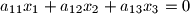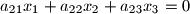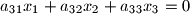with unknowns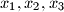$x_1, x_2, x_3$. The coefficients satisfy the conditions:

a)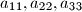$a_{11}, a_{22}, a_{33}$ are positive numbers;

b) the remaining coefficients are negative numbers;

c) in each equation, the sum ofthe coefficients is positive.

Prove that the given system has only the solution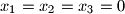$x_1=x_2=x_3=0$.
Source: Međunarodna matematička olimpijada, shortlist 1965